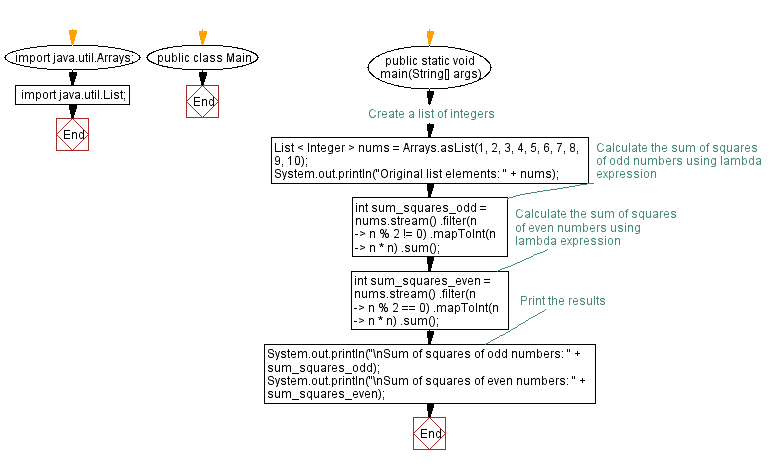# Java program to calculate sum of squares of odd and even numbers using lambda expression

## Java Lambda Program: Exercise-15 with Solution

Write a Java program to implement a lambda expression to calculate the sum of squares of all odd and even numbers in a list.

Sample Solution:

Java Code:

``````import java.util.Arrays;
import java.util.List;

public class Main {
public static void main(String[] args) {
// Create a list of integers
List < Integer > nums = Arrays.asList(1, 2, 3, 4, 5, 6, 7, 8, 9, 10);
System.out.println("Original list elements: " + nums);
// Calculate the sum of squares of odd numbers using lambda expression
int sum_squares_odd = nums.stream()
.filter(n -> n % 2 != 0)
.mapToInt(n -> n * n)
.sum();

// Calculate the sum of squares of even numbers using lambda expression
int sum_squares_even = nums.stream()
.filter(n -> n % 2 == 0)
.mapToInt(n -> n * n)
.sum();

// Print the results
System.out.println("\nSum of squares of odd numbers: " + sum_squares_odd);
System.out.println("\nSum of squares of even numbers: " + sum_squares_even);
}
}
``````

Sample Output:

```Original list elements: [1, 2, 3, 4, 5, 6, 7, 8, 9, 10]

Sum of squares of odd numbers: 165

Sum of squares of even numbers: 220
```

Explanation:

The necessary classes are imported: Arrays and Lists.

In the main method, we create a list of integers called nums using Arrays.asList().

A lambda expression is used to calculate the sum of squares of odd numbers:

• Use the stream() method to create a stream of integers from the nums list.
• Use the filter() method to filter out odd numbers by checking if the remainder of dividing the number by 2 is not equal to 0.
• Use the mapToInt() method to square each odd number.
• Use the sum() method to calculate the sum of squares of odd numbers.
• Store the result in the variable sum_squares_odd.

A similar lambda expression is used to calculate the sum of squares of even numbers:

• Use the filter() method to filter out even numbers by checking if the remainder of dividing the number by 2 is equal to 0.
• Store the result in the variable sum_squares_even.

Finally, System.out.println() function prints the result.

Flowchart:Live Demo:

Java Code Editor:

Improve this sample solution and post your code through Disqus

Java Lambda Exercises Previous: Java program to check Palindrome string using lambda expression.
Java Lambda Exercises Next: Java program to check if a list contains a specific word using lambda expression.

What is the difficulty level of this exercise?

Test your Programming skills with w3resource's quiz.

﻿